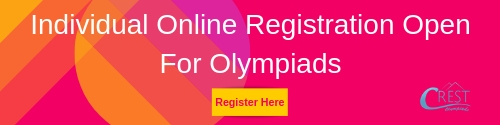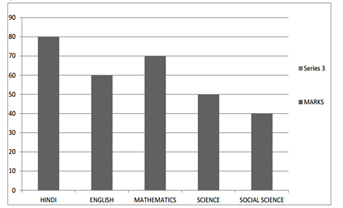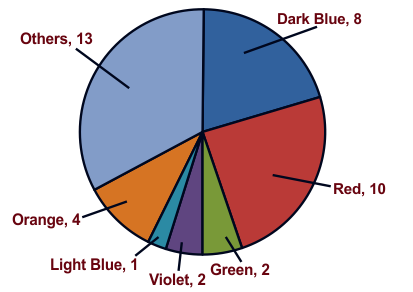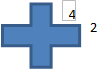ASSET - Math PDF Sample Papers for Class 6

Resources:

Class 6 sample paper & practice questions for ASSET Math are given below. Syllabus for level 1 is also mentioned for these exams. You can refer these sample paper & quiz for preparing for the exam.Sample Questions from Olympiad Success:

 Q.1 Q.2 Q.3 Q.4 Q.5 Q.6 Q.7 Q.8 Q.9 Q.10
 Q.1 The following bar graph shows the marks obtained by Ramesh in different subjects in the Session Ending Examination-2012. Which of the following is the difference between the maximum & the minimum marks?a) 40 b) 30 c) 20 d) 10
 Q.2 Javed was given 5/7 of a basket of oranges. What fraction of oranges was left in the basket? a) 4/7 b) 5/7 c) 2/7 d) 12/7
 Q.3 The two consecutive integers between which the fraction 5/7 lies are: a) 5 and 6 b) 0 and 1 c) 5 and 7 d) 6 and 7
 Q.4 3 X 10000 + 7 X 1000 + 9 X 100 + 0 X 10 + 4 is the same as: a) 37914 b) 37940 c) 37904 d) 379409
 Q.5 The C.P. of 25 articles is equal to the S.P of 20 articles. What is the gain percentage? a) 25% b) 20% c) 30% d) 50%
 Q.6 How many prime factors do 1256 has? a) 2 b) 8 c) 3 d) 4
 Q.7 What is the value of 81265 x 169 - 81265 x 69? a) 8126500 b) 81265000 c) Zero d) 100
 Q.8 There are 100 teachers in a school for 3000 students. Which of the following is the teacher student ratio? a) 3:100 b) 1:30 c) 1:1 d) 30:1
 Q.9 Which of these are favourites of equal number of kids?a) Red and Orange b) Red and Dark Blue c) Violet and Light Blue d) Violet and Green
 Q.10 Find the perimeter.a) 42 b) 44 c) 40 d) 46Sample PDF of ASSET - ASSET Math PDF Sample Papers (MATH) for Class 6: